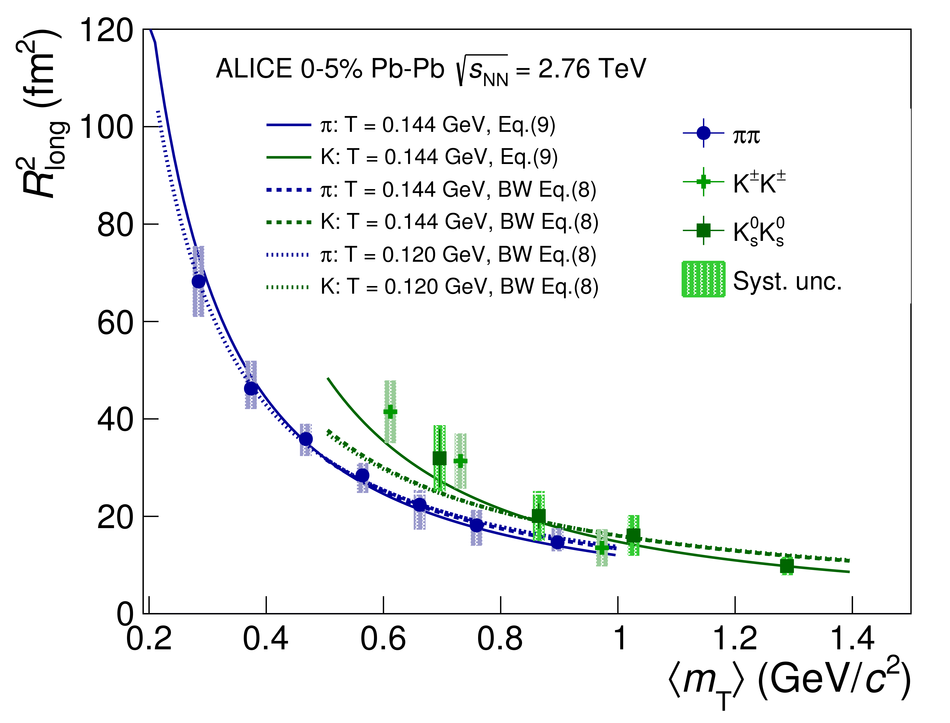# Kaon femtoscopy in Pb-Pb collisions at $\sqrt{s_{\rm{NN}}}$ = 2.76 TeV

We present the results of three-dimensional femtoscopic analyses for charged and neutral kaons recorded by ALICE in Pb-Pb collisions at $\sqrt{s_{\rm{NN}}}$ = 2.76 TeV. Femtoscopy is used to measure the space-time characteristics of particle production from the effects of quantum statistics and final-state interactions in two-particle correlations. Kaon femtoscopy is an important supplement to that of pions because it allows one to distinguish between different model scenarios working equally well for pions. In particular, we compare the measured 3D kaon radii with a purely hydrodynamical calculation and a model where the hydrodynamic phase is followed by a hadronic rescattering stage. The former predicts an approximate transverse mass ($m_{\mathrm{T}}$) scaling of source radii obtained from pion and kaon correlations. This $m_{\mathrm{T}}$ scaling appears to be broken in our data, which indicates the importance of the hadronic rescattering phase at LHC energies. A $k_{\mathrm{T}}$ scaling of pion and kaon source radii is observed instead. The time of maximal emission of the system is estimated using the three-dimensional femtoscopic analysis for kaons. The measured emission time is larger than that of pions. Our observation is well supported by the hydrokinetic model predictions.

Figures

## Figure 1

 Single $\Kpm$ purity (a) and pair purity for small relative momenta (b) for different centralities. In (b) the $k_{\rm T}$ values for different centrality intervals are slightly offset for clarity.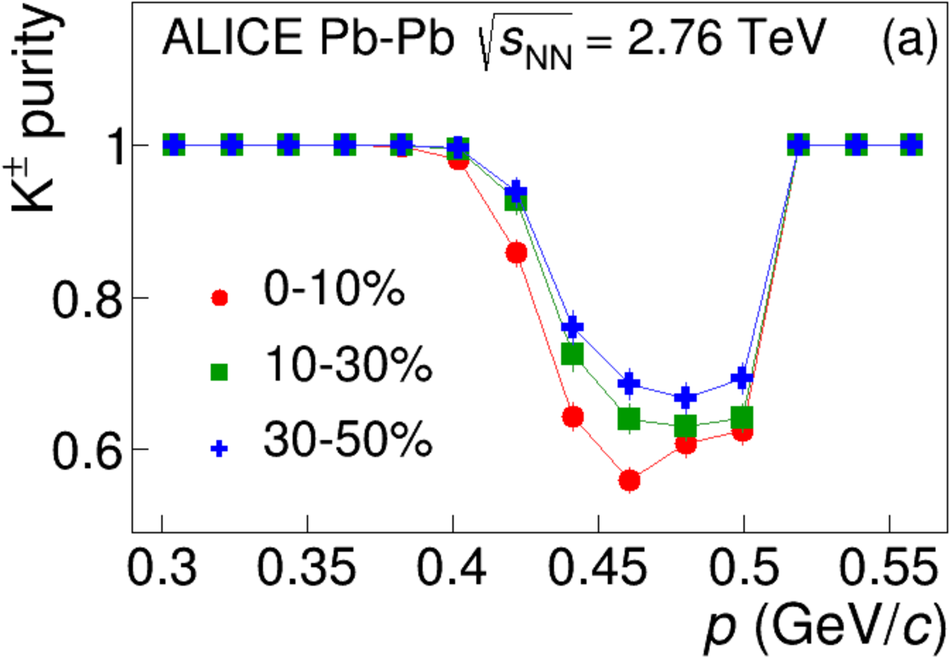## Figure 2

 A sample projected $\Kpm\Kpm$ correlation function with fit. The error bars are statistical only. Systematic uncertainties on the points are equal to or less than the statistical error bars shown.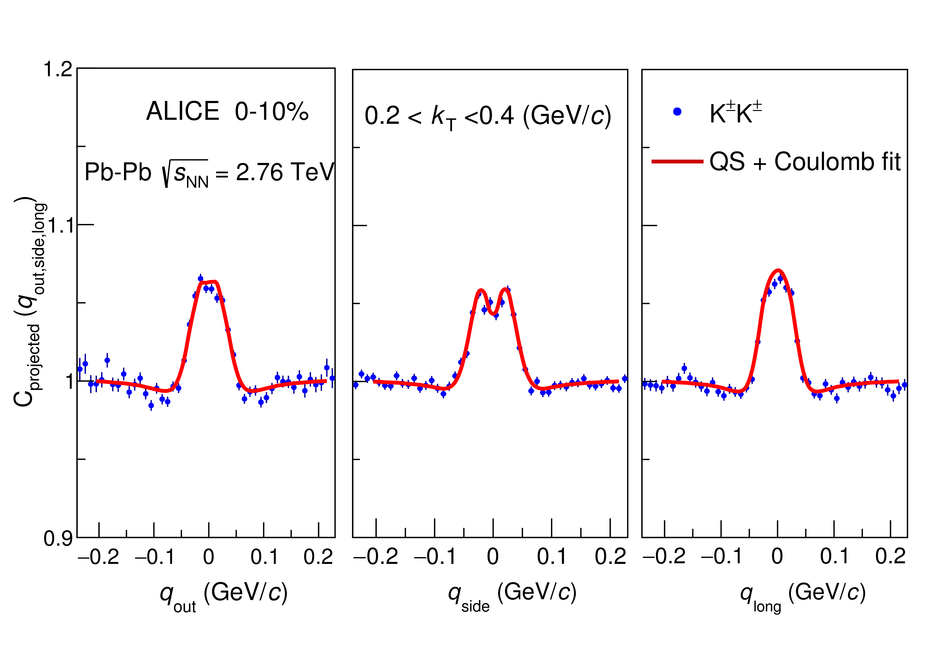## Figure 3

 A sample projected $\Kzs\Kzs$ correlation function with fit. Also shown is the contribution to the fit from the quantum statistics part only. The error bars are statistical only. Systematic uncertainties on the points are equal to or less than the statistical error bars shown.## Figure 4

 The 3D LCMS radii vs. $m_{\mathrm{T}}$ for charged (light green crosses) and neutral (dark green squares) kaons and pions (blue circles) in comparison withthe theoretical predictions of the (3+1)D Hydro + THERMINATOR-2 model for pions (blue solid lines) and kaons (red solid lines).## Figure 5

 The 3D LCMS radii vs. $m_{\mathrm{T}}$for 0--5\% most central collisionsin comparison with the theoretical predictions of HKM for pions (blue lines) and kaons (red lines)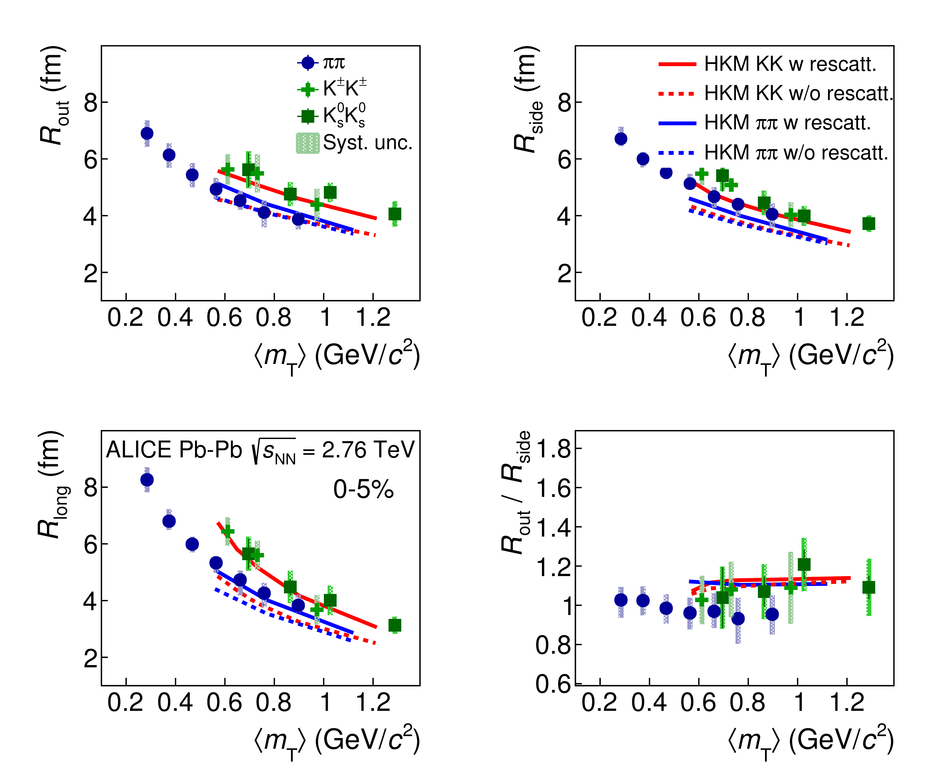## Figure 6

 The 3D LCMS radii vs. $k_{\mathrm{T}}$ for charged (light green crosses) and neutral (dark green squares) kaons and pions (blue circles)## Figure 7

 $R_{\rm out}/R_{\rm side}$ vs. $m_{\mathrm{T}}$ for pions and kaonsfor different centrality intervals.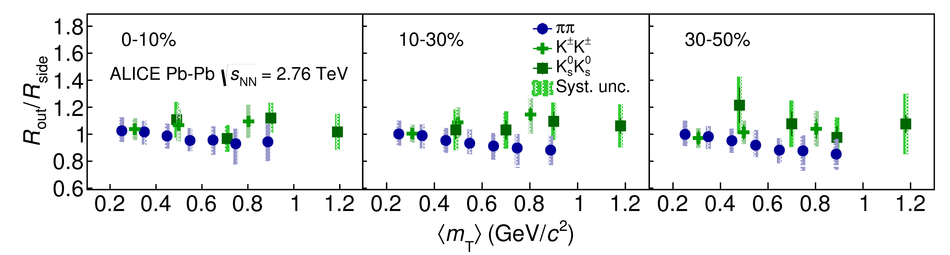## Figure 8

 $R^{2}_{\rm long}$ vs. $m_{\mathrm{T}}$ for kaons and pions The solid lines show the fit using Eq.~(\ref{eq:tau_YS})for pions and kaons to extract the emission times ($\tau$); the dashed and dotted linesshow the fit using Eq.~(\ref{eq:tau_stand}) with $T_{\rm kin}$ = 0.144~GeV and $T_{\rm kin}$ = 0.120~GeV, respectively. For pions at small $m_{\mathrm{T}}$, the dashed and dotted line coincide